Open in App
Not now

# ML | Linear Regression

• Difficulty Level : Medium
• Last Updated : 16 Jan, 2023

Linear Regression is a machine learning algorithm based on supervised learning. It performs a regression task. Regression models a target prediction value based on independent variables. It is mostly used for finding out the relationship between variables and forecasting. Different regression models differ based on – the kind of relationship between dependent and independent variables they are considering, and the number of independent variables getting used. There are many names for a regression’s dependent variable.  It may be called an outcome variable, criterion variable, endogenous variable, or regressand.  The independent variables can be called exogenous variables, predictor variables, or regressors.

Linear regression is used in many different fields, including finance, economics, and psychology, to understand and predict the behavior of a particular variable. For example, in finance, linear regression might be used to understand the relationship between a company’s stock price and its earnings, or to predict the future value of a currency based on its past performance.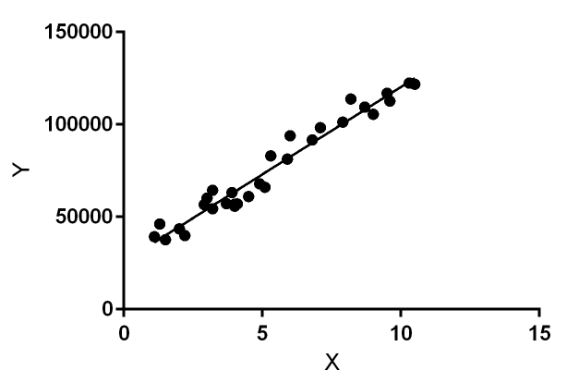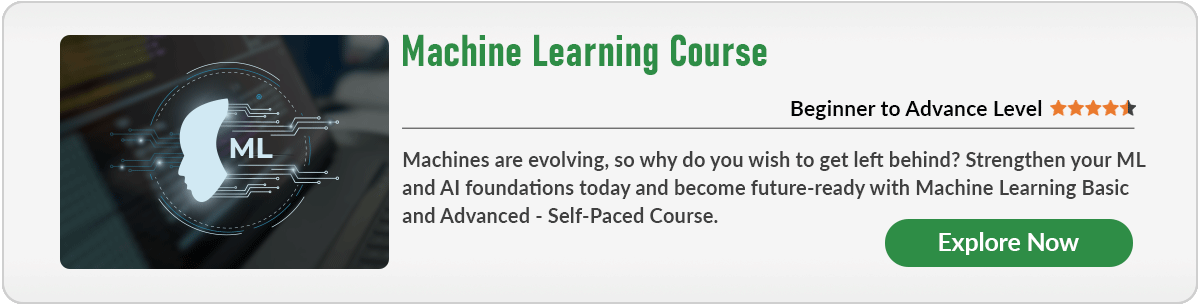Linear regression performs the task to predict a dependent variable value (y) based on a given independent variable (x)). Hence, the name is Linear Regression. In the figure above, X (input) is the work experience and Y (output) is the salary of a person. The regression line is the best fit line for our model. Hypothesis function for Linear Regression :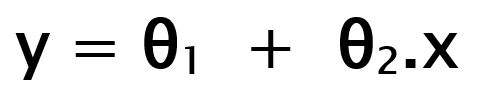While training the model we are given : x: input training data (univariate – one input variable(parameter)) y: labels to data (Supervised learning) When training the model – it fits the best line to predict the value of y for a given value of x. The model gets the best regression fit line by finding the best θ1 and θ2 values. θ1: intercept θ2: coefficient of x Once we find the best θ1 and θ2 values, we get the best fit line. So when we are finally using our model for prediction, it will predict the value of y for the input value of x. How to update θ1 and θ2 values to get the best fit line ?

Linear regression is a powerful tool for understanding and predicting the behavior of a variable, but it has some limitations. One limitation is that it assumes a linear relationship between the independent variables and the dependent variable, which may not always be the case. In addition, linear regression is sensitive to outliers, or data points that are significantly different from the rest of the data. These outliers can have a disproportionate effect on the fitted line, leading to inaccurate predictions.Cost Function (J): By achieving the best-fit regression line, the model aims to predict y value such that the error difference between predicted value and true value is minimum. So, it is very important to update the θ1 and θ2 values, to reach the best value that minimize the error between predicted y value (pred) and true y value (y).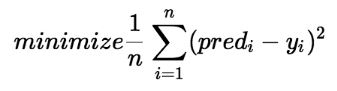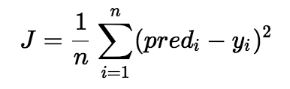Cost function(J) of Linear Regression is the Root Mean Squared Error (RMSE) between predicted y value (pred) and true y value (y). Gradient Descent: To update θ1 and θ2 values in order to reduce Cost function (minimizing RMSE value) and achieving the best fit line the model uses Gradient Descent. The idea is to start with random θ1 and θ2 values and then iteratively updating the values, reaching minimum cost.

My Personal Notes arrow_drop_up